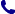• Kontakt
• Newsletter Zapisz się po informacje o nowościach i specjalnych ofertach. Administrator danych: ABE-IPS sp. z o.o.
Wybierz interesujące Cię kategorie
Wyrażam zgodę na przetwarzanie moich danych osobowych w celach marketingowych, tym samym akceptuję Regulamin Newslettera i Politykę prywatności.
Wyrażam zgodę na przesyłanie informacji handlowych drogą elektroniczną.
Wypisz się
• Klienci instytucjonalni
• Dostawa
• O nas
Dostęp on-line

Książki

0.00 PLN
Schowek (0)
 Schowek jest pusty

A Study in Derived Algebraic Geometry

 Autorzy Wydawnictwo American Mathematical Society Data wydania 01/09/2020 Wydanie Pierwsze Liczba stron 436 Forma publikacji książka w miękkiej oprawie Poziom zaawansowania Dla profesjonalistów, specjalistów i badaczy naukowych Język angielski ISBN 9781470452858 Kategorie Algebra, Geometria algebraiczna
502.00 PLN (z VAT)
\$125.65 / €110.81 / £92.60 /
Produkt dostępny
Dostawa 2 dni
Ilość
Do schowka

Opis książki

Derived algebraic geometry is a far-reaching generalization of algebraic geometry. It has found numerous applications in other parts of mathematics, most prominently in representation theory. This volume develops deformation theory, Lie theory and the theory of algebroids in the context of derived algebraic geometry. To that end, it introduces the notion of inf-scheme, which is an infinitesimal deformation of a scheme and studies ind-coherent sheaves on such. As an application of the general theory, the six-functor formalism for D-modules in derived geometry is obtained. This volume consists of two parts. The first part introduces the notion of ind-scheme and extends the theory of ind-coherent sheaves to inf-schemes, obtaining the theory of D-modules as an application. The second part establishes the equivalence between formal Lie group(oids) and Lie algebr(oids) in the category of ind-coherent sheaves. This equivalence gives a vast generalization of the equivalence between Lie algebras and formal moduli problems. This theory is applied to study natural filtrations in formal derived geometry generalizing the Hodge filtration.

A Study in Derived Algebraic Geometry

Spis treści

Inf-schemes: Introduction
Deformation theory
Ind-schemes and inf-schemes
Ind-coherent sheaves on ind-inf-schemes
An application: Crystals
Formal geometry: Introduction
Formal moduli
Lie algebras and co-commutative co-algebras
Formal groups and Lie algebras
Lie algebroids
Infinitesimal differential geometry
Bibliography
Index of notations
Index.

Polecamy również książki801 777 223